This document contains R versions of the boxed examples from **Chapter 3} of the “Analysis and Interpretation of Freshwater Fisheries Data” book. Some sections build on descriptions from previous sections, so each section may not stand completely on its own. More thorough discussions of the following items are available in linked vignettes:

The following additional packages are required to complete all of the examples (with the required functions noted as a comment and also noted in the specific examples below).

> library(FSA)       # Summarize, fitPlot, binCI, view
> library(NCStats)   # sdCalc
> library(arm)       # se.ranef
> library(car)       # Anova, leveneTest, outlierTest
> library(languageR) # pvals.fnc
> library(lattice)   # bwplot, densityplot
> library(lme4)      # lmer and related extractors
> library(lsmeans)   # lsmeans
> library(sciplot)   # se
> library(survey)    # svydesign, svymean

In addition, external tab-delimited text files are used to hold the data required for each example. These data are loaded into R in each example with read.table(). Before using read.table() the working directory of R must be set to where these files are located on your computer. The working directory for all data files on my computer is set with

> setwd("c:/aaaWork/web/fishR/BookVignettes/AIFFD/")

I also prefer to not show significance stars for hypothesis test output and set contrasts in such a manner as to force R output to match SAS output for linear model summaries. These options are set below.

> options(show.signif.stars=FALSE,contrasts=c("contr.sum","contr.poly"))

## 3.1 Example of Estimating the Mean Based on Simple Random Sampling

Fifteen sites were randomly selected from an X-Y grid superimposed on a shallow lake. At each site, the catch of central mudminnow (Umbra limi) in a throw trap (assumed to be equally efficient at all sites in the lake) was recorded. The goal of the sampling was to determine the mean density of central mudminnows in the lake.

### 3.1.1 Preparing Data

The box3_1.txt is read and the structure of the data frame is observed.

> d1 <- read.table("data/box3_1.txt",header=TRUE)
> str(d1)
'data.frame':   15 obs. of  1 variable:
$catch: int 3 0 4 1 4 1 1 0 1 0 ... ### 3.1.2 Compute Summary Statistics The mean and standard deviation for the quantitative variable is computed with Summarize() from the FSA package. The single required argument is the vector containing the quantitative data. > Summarize(d1$catch)
        n    nvalid      mean        sd       min        Q1    median        Q3
15.000000 15.000000  1.600000  1.404076  0.000000  0.500000  1.000000  2.500000
max  percZero
4.000000 26.666667 

If you do not want to load the FSA package, then the same calculations are made with summary() and sd() of the R base packages.

> summary(d1$catch)  Min. 1st Qu. Median Mean 3rd Qu. Max. 0.0 0.5 1.0 1.6 2.5 4.0  > sd(d1$catch)
 1.404076

Regardless of which way you summarize the results, you can compute the standard error with se() from the sciplot package.

> se(d1$catch)  0.3625308 ### 3.1.3 Constructing Confidence Intervals for the Population Mean The critical t value for computing a confidence interval is found with qt(). This function requires an upper-tail probability (i.e., $$\frac{1-C}{2}$$ where $$C$$ is the level of confidence), the degrees-of-freedom (which uses length() with the vector of data as the only argument to find $$n$$), and the lower.tail=FALSE argument. > df <- length(d1$catch)-1             # compute df as n-1
> C <- 0.95                            # set confidence level
> a2 <- (1-C)/2                        # find upper tail probability
> ( ct <- qt(a2,df,lower.tail=FALSE) ) # find critical t-value
 2.144787

The two endpoints of the confidence interval can then be calculated with the mean (using mean() with the vector of data as the only argument), standard error, and the critical t value.

> ( mn <- mean(d1$catch) ) # find (and save) the mean  1.6 > ( semn <- se(d1$catch) )              # find (and save) the SE of the mean
 0.3625308
> ( LCI <- mn-ct*semn )
 0.8224488
> ( UCI <- mn+ct*semn )
 2.377551

The confidence interval, along with a great deal of other information, is computed from the raw data with t.test(). In this simplest form, this function only requires the vector of quantitative data as the first argument and an optional level of confidence, as a proportion, in the conf.level= argument (default is 0.95).

> t.test(d1$catch) One Sample t-test with d1$catch
t = 4.4134, df = 14, p-value = 0.0005894
alternative hypothesis: true mean is not equal to 0
95 percent confidence interval:
0.8224488 2.3775512
sample estimates:
mean of x
1.6 

### 3.1.4 Demonstration of Standard Deviation Calculation

The intermediate steps in computing a standard deviation, as shown at the beginning of Box 3.1, are demonstrated with sdCalc() from the NCStats package.

> ( p <- d2$n/ttl ) # find proportion of "successes"  0.47413793 0.18965517 0.08620690 0.15517241 0.05172414 0.02586207 0.00862069  0.00862069 The standard errors are then computed with $$\sqrt{\frac{p*(1-p)}{n-1}}$$ (note that the text defines $$q=1-p$$). > ( seps <- sqrt(p*(1-p)/(ttl-1)) )  0.04656283 0.03655682 0.02617255 0.03376311 0.02065214 0.01480106 0.00862069  0.00862069 ### 3.2.3 Confidence Intervals for Population Proportions I The critical value from the F distribution is found with qf(). This function requires an upper-tail probability as the first argument, numerator df as the second argument, denominator df as the third argument, and lower.tail=FALSE. For example, the two critical F values used for only the first age-group in Box 3.2 are found below. > alpha <- 0.05 > n <- ttl > a <- 55 # demonstrate for first group only > ( f.lwr <- qf(alpha,2*(n-a+1),2*a,lower.tail=FALSE) )  1.36009 > ( f.upr <- qf(alpha,2*a+2,2*(n-a+1)-2,lower.tail=FALSE) )  1.355947 The CIs for the first age-group can then be computed with the formulas shown in the text. > ( LCI <- a / (a+(n-a+1)*f.lwr) )  0.3947589 > ( UCI <- ((a+1)*f.upr)/(n-a+(a+1)*f.upr) )  0.5545268 Vector arithmetic in R can then be used to efficiently compute t CIs for all of the age-groups. > LCIs <- d2$n/(d2$n+(ttl-d2$n+1)*qf(alpha,2*(ttl-d2$n+1),2*d2$n,lower.tail=FALSE))
> UCIs <- ((d2$n+1)*qf(alpha,2*d2$n+2,2*(ttl-d2$n+1)-2,lower.tail=FALSE))/ (ttl-d2$n+(d2$n+1)*qf(alpha,2*d2$n+2,2*(ttl-d2$n+1)-2,lower.tail=FALSE)) > data.frame(age=d2$age,p,seps,LCIs,UCIs)               # put in data frame just to show
  age          p       seps         LCIs       UCIs
1   0 0.47413793 0.04656283 0.3947588631 0.55452678
2   1 0.18965517 0.03655682 0.1320279845 0.25960996
3   2 0.08620690 0.02617255 0.0475164835 0.14183827
4   3 0.15517241 0.03376311 0.1027583277 0.22137272
5   4 0.05172414 0.02065214 0.0227626672 0.09953204
6   5 0.02586207 0.01480106 0.0070853559 0.06548328
7   6 0.00862069 0.00862069 0.0004420858 0.04024133
8   7 0.00862069 0.00862069 0.0004420858 0.04024133

### 3.2.4 Confidence Intervals for Population Proportions II

Many authors suggest methods for computing confidence intervals for proportions other than using the F distribution as shown in the text. One such method is the so-called “Wilson” method which is the default method in binCI() from the FSA package. This function requires the “number of success” as the first argument, the “number of trials” as the second argument, and an optional level of confidence as a proportion (defaults to 0.95) in conf.level=.

> binCI(d2$n,ttl)  95% LCI 95% UCI 0.3855640505 0.56436980 0.1287138187 0.27049243 0.0474993548 0.15144231 0.1004660814 0.23198530 0.0239186535 0.10826815 0.0088338879 0.07328676 0.0004421836 0.04721983 0.0004421836 0.04721983 ## 3.3 Example of Estimating a Ratio in Simple Random Sampling Twenty yellow perch (Perca flavescens) were randomly sampled from a lake, and weights of zooplankton, benthos, and fish in each yellow perch stomach were measured. The goal was to determine the ratio of each prey category to total weight of prey in the diet of the yellow perch population. ### 3.3.1 Preparing Data The box3_3.txt is read, the structure of the data frame, and random rows of the data is observed below. In addition, the table shown in Box 3.3 and the methods below require a column that consists of the total prey weight. This column is appended to the data frame and a random six rows of the data frame are observed with view() from the FSA package to assure that this computation makes sense. > d3 <- read.table("data/box3_3.txt",header=TRUE) > str(d3) 'data.frame': 20 obs. of 3 variables:$ Zooplankton: num  0 0.2 0.593 0.356 0.07 0.191 0.012 0.017 0.4 0.202 ...
$Benthos : num 0 2.501 0.054 0.741 1.112 ...$ Fish       : num  16.2 0 0 0 0 ...
> d3$ttlW <- d3$Zooplankton + d3$Benthos + d3$Fish
> view(d3)
   Zooplankton Benthos Fish  ttlW
6        0.191   1.734    0 1.925
7        0.012   0.022    0 0.034
8        0.017   2.822    0 2.839
9        0.400   2.796    0 3.196
11       0.591   0.559    0 1.150
17       0.937   0.354    0 1.291

### 3.3.2 Computing the Ratio Estimators

The ratio estimators can be computed in R with the help of svydesign() and svyratio(), both of which are from the survey package. The svydesign() function is used to identify the design of sample. In the case of simple random sample as in this example, this function only requires the id=~1 as the first argument (essentially identifying that each row of the data frame is a randomly sampled primary sampling unit) and the appropriate data frame in data=. The ratio estimate is then computed with svyratio() with the variable to serve as the numerator as a “formula” in the first argument, the variable to serve as the denominator as a “formula” in the second argument, the svydesign() as the third argument. The corresponding confidence interval is computed by submitting the saved svyratio() object to confint().

> srs <- svydesign(id=~1,data=d3)
Warning in svydesign.default(id = ~1, data = d3): No weights or probabilities
supplied, assuming equal probability
> ( res1 <- svyratio(~Zooplankton,~ttlW,srs) )
Ratio estimator: svyratio.survey.design2(~Zooplankton, ~ttlW, srs)
Ratios=
ttlW
Zooplankton 0.1272082
SEs=
ttlW
Zooplankton 0.05484727
> confint(res1)
                     2.5 %    97.5 %
Zooplankton/ttlW 0.0197095 0.2347068

For efficiency, if the numerator argument is the apparent “sum” of a number of variables, then the ratio estimators will be computed for each of those variables.

> ( res2 <- svyratio(~Zooplankton+Benthos+Fish,~ttlW,srs) )
Ratio estimator: svyratio.survey.design2(~Zooplankton + Benthos + Fish, ~ttlW,
srs)
Ratios=
ttlW
Zooplankton 0.1272082
Benthos     0.3727388
Fish        0.5000530
SEs=
ttlW
Zooplankton 0.05484727
Benthos     0.15230946
Fish        0.19604042
> confint(res2)
                      2.5 %    97.5 %
Zooplankton/ttlW 0.01970950 0.2347068
Benthos/ttlW     0.07421778 0.6712599
Fish/ttlW        0.11582083 0.8842852

The “formula” arguments in svydesign() MUST begin with a tilde.

## 3.4 Example of Stratified Random Sampling

A grid was superimposed on the map of a shallow lake, and all grid cells were classified as being in one of three depth strata (0-2m, 2-4m, >4m). Ten grid cells were sampled in each depth stratum, and at each site the catch of age-0 yellow perch (Perca flavescens) in a throw trap (assumed to be equally efficient at all sites in the lake) was recorded. The goal of the sampling program was to estimate the mean density of age-0 yellow perch.

### 3.4.1 Preparing Data

The box3_4.txt is read, the structure of the data frame, and six random rows of the data are observed below. Note that cells contains the number of grid cells in each stratum and this value is repeated for each row of a particular stratum.

> d4 <- read.table("data/box3_4.txt",header=TRUE)
> str(d4)
'data.frame':   30 obs. of  3 variables:
$stratum: Factor w/ 3 levels ">4m","0-2m","2-4m": 2 2 2 2 2 2 2 2 2 2 ...$ catch  : int  0 2 2 2 3 1 3 2 2 0 ...
$cells : int 172 172 172 172 172 172 172 172 172 172 ... > view(d4)  stratum catch cells 3 0-2m 2 172 4 0-2m 2 172 13 2-4m 3 80 16 2-4m 4 80 19 2-4m 2 80 21 >4m 7 68 ### 3.4.2 Computing Mean Across All Strata The survey package provides a simple methodology to compute the overall mean with standard error (SE) and confidence interval from a stratified design. This methodology requires the user to first identify characteristics about the study design with svydesign(). For the methodology in Box 3.4, svydesign() requires four arguments as follows: • id: a “formula” that indicates which variable identifies the primary sampling unit. If set to ~1 then each row in the data frame is a primary sampling unit. • strata: a “formula”, usually of the form ~stratavar where stratavar is a variable that indicates to which stratum a sampling unit belongs. • fpc: a “formula”, usually of the form ~nvar where nvar is a variable that indicates the number of sampling units in each stratum. • data: the data frame containing the data. The results of svydesign() should be saved to an object and a summary of this new object is obtained with summary(). > dstrat <- svydesign(id=~1,strata=~stratum,fpc=~cells,data=d4) > summary(dstrat) Stratified Independent Sampling design svydesign(id = ~1, strata = ~stratum, fpc = ~cells, data = d4) Probabilities: Min. 1st Qu. Median Mean 3rd Qu. Max. 0.05814 0.05814 0.12500 0.11010 0.14710 0.14710 Stratum Sizes: >4m 0-2m 2-4m obs 10 10 10 design.PSU 10 10 10 actual.PSU 10 10 10 Population stratum sizes (PSUs): >4m 0-2m 2-4m 68 172 80 Data variables:  "stratum" "catch" "cells"  The “formula” arguments in svydesign() MUST begin with a tilde. The overall mean and SE from the design in the svydesign() object is computed with svymean(). This function takes a “formula”, usually of the form ~quantvar where quantvar is the variable for which the mean should be computed, as the first argument and the saved svydesign object as the second argument. The svymean() function will return the mean and SE when printed. A confidence interval for the overall mean is computed by saving the result of svymean() to an object and submitting it to confint(). > ( d.mns <- svymean(~catch,dstrat) )  mean SE catch 2.85 0.2128 > confint(d.mns)  2.5 % 97.5 % catch 2.433005 3.266995 ### 3.4.3 Additional Functionality The survey package also provides functions for extracting the coefficient of variation (i.e., use cv()) and the so-called “design effect” (add deff=TRUE to svymean()), which is the ratio of the variance using the stratified design to the variance as if a simple random sample had been used. > cv(d.mns)  catch catch 0.07465136 > svymean(~catch,dstrat,deff=TRUE)  mean SE DEff catch 2.85000 0.21276 0.3974 ## 3.5 Example of Cluster Sampling Five throw nets were deployed at random locations along the shoreline of a lake to collect age=0 bluegill (Lepomis macrochirus). Greater sampling effort would usually be required, but data from these five nets are used to illustrate the procedure. The weight (g) of each of the age-0 bluegill was measured. The goal of sampling was to estimate the mean biomass of age-0 bluegill per net, the mean catch per net, and the mean weight of individual age-0 bluegill. ### 3.5.1 Preparing Data The box3_5.txt is read, the structure of the data frame, and six random rows of the data are observed below. Note that catch1 contains the total number of fish caught in a net in the first example in Box 3.5 (where all fish captured were measured). In contrast, catch2 is the total number of fish caught in a net in the second sample (where only a subsample of fish captured were measured). Also note that net is a “grouping variable” and is changed to a factor in R. Finally, note that fish is the fish identification number within a net. > d5 <- read.table("data/box3_5.txt",header=TRUE) > str(d5) 'data.frame': 26 obs. of 5 variables:$ net   : int  1 1 1 1 1 1 1 1 1 1 ...
$fish : int 1 2 3 4 5 6 7 8 9 10 ...$ weight: num  0.495 0.391 0.274 0.47 0.309 0.369 0.381 0.308 0.42 0.326 ...
$catch1: int 10 10 10 10 10 10 10 10 10 10 ...$ catch2: int  48 48 48 48 48 48 48 48 48 48 ...
> d5$net <- factor(d5$net)
> view(d5)
   net fish weight catch1 catch2
6    1    6  0.369     10     48
11   2    1  0.319      5      5
12   2    2  0.419      5      5
13   2    3  0.503      5      5
17   3    2  0.497      7     20
26   5    3  0.681      3      3

### 3.5.2 Single-Stage Cluster Sample

The total catch and total weight of fish caught are computed with the aid of tapply(). The tapply() function is used to apply a function (given in the FUN= argument) to the quantitative variable given in the first argument to each individual in groups defined by the group factor variable in the second argument. Thus, the mean catch per net (really the total catch in each net because the catch per net was repeated for each fish caught in that net) and the total (sum) weight of all fish caught in each net are computed as shown below.

> ( catch <- tapply(d5$catch1,d5$net,FUN=mean) )
 1  2  3  4  5
10  5  7  0  3 
> ( ttl.wt <- tapply(d5$weight,d5$net,FUN=sum) )
    1     2     3     4     5
3.743 2.183 3.290    NA 1.863 

The mean fish weight is computed with the aid of svydesign() and svymean(), both of which are from the survey package, and were also described BOX 3.4. The “design” of the sampling scheme is declared in svydesign(). The id= argument is a formula used to identify which variable indicates a primary sampling unit (PSU). In this case, the primary sampling unit is a net (as stored in the net variable). As the total number of PSU are not know in this case, the fpc= argument (Which was described in BOX 3.4). is not used. Finally, the data frame containing the variables is supplied in data=. The mean weight is then computed with svymean() with the weight variable in a formula as the first argument and the svydesign object as the second argument. Because there is an NA in the weight variable corresponding to no fish being caught in the fourth net, the na.rm=TRUE argument must be used to tell R to “remove” or “ignore” the NAs. The saved svymean object is submitted to confint() to construct a confidence interval for the mean.

> dclust <- svydesign(id=~net,data=d5)
Warning in svydesign.default(id = ~net, data = d5): No weights or probabilities
supplied, assuming equal probability
> summary(dclust)
1 - level Cluster Sampling design (with replacement)
With (5) clusters.
svydesign(id = ~net, data = d5)
Probabilities:
Min. 1st Qu.  Median    Mean 3rd Qu.    Max.
1       1       1       1       1       1
Data variables:
 "net"    "fish"   "weight" "catch1" "catch2"
> ( mn.wt <- svymean(~weight,dclust,na.rm=TRUE) )
          mean     SE
weight 0.44316 0.0399
> confint(mn.wt)
           2.5 %    97.5 %
weight 0.3649976 0.5213224

The confidence intervals in this example do not match those in Box 3.5 in the text. As the mean and SE are the same as those in the text, the discrepancy must be in the use of the critical t value. I could not replicate nor find a reasoning for the critical t used in the text.

### 3.5.3 Two-Stage Cluster Sample

I have not yet determined how to do this analysis without simply hard-coding the formulas.

## 3.6 Example of Systematic Sampling with Two Starting Points

The width of a stream was measured at sampling locations arranged every 20 m from two random starting points, with 15 points sampled for each random starting point.

### 3.6.1 Preparing Data

The box3_6.txt is read and the structure of the data frame is observed below.

> d6 <- read.table("data/box3_6.txt",header=TRUE)
> str(d6)
'data.frame':   30 obs. of  3 variables:
$start : int 1 1 1 1 1 1 1 1 1 1 ...$ distUp: int  3 23 43 63 83 103 123 143 163 183 ...
$width : num 6.1 11.4 13.7 11.3 11.7 13.3 12.1 11.5 6.4 34.8 ... As described in the text, a systematic sample with two random starting points can be analyzed as a single-stage cluster sample which was described in BOX 3.5. The methods of BOX 3.5 are implemented below with the id= argument set equal to start which defines the samples corresponding to the two starting points. > dsyst <- svydesign(id=~start,data=d6) Warning in svydesign.default(id = ~start, data = d6): No weights or probabilities supplied, assuming equal probability > summary(dsyst) 1 - level Cluster Sampling design (with replacement) With (2) clusters. svydesign(id = ~start, data = d6) Probabilities: Min. 1st Qu. Median Mean 3rd Qu. Max. 1 1 1 1 1 1 Data variables:  "start" "distUp" "width"  > ( mn.wid <- svymean(~width,dsyst) )  mean SE width 16.307 0.5 > confint(mn.wt)  2.5 % 97.5 % weight 0.3649976 0.5213224 ## 3.7 Example of Regression or Double Sampling The percent of coverage by woody material was visually estimated at 25 randomly selected points along a stream, and the actual amount of woody material coverage was measured at 10 of these points, with the goal of estimating mean woody material coverage for this reach. The regression between the visually estimated coverage and the measured coverage gave the following equation – Measured coverage = 7.2937 + 1.0357(estimated coverage). ### 3.7.1 Preparing Data The box3_7.txt is read and the structure of the data frame is observed below. A new data frame that contains only those observations where both an estimated and a measured variable were recorded was constructed. > d7 <- read.table("data/box3_7.txt",header=TRUE) > str(d7) 'data.frame': 25 obs. of 2 variables:$ estimated: int  30 40 60 20 20 20 30 0 40 50 ...
$measured : int 34 52 66 26 21 25 38 14 54 64 ... > d7a <- d7[!is.na(d7$measured),]
> str(d7a)
'data.frame':   10 obs. of  2 variables:
$estimated: int 30 40 60 20 20 20 30 0 40 50$ measured : int  34 52 66 26 21 25 38 14 54 64

### 3.7.2 Fitting the Regression

The regression and calculation shown in Box 3.7 can be simplified by “centering” the estimated variable. A variable is “centered” by subtracting the mean of that variable. The value of centering is that the intercept when the centered variable is used is equal to the mean of the response variable (i.e., measured). The slope is unaffected by using the centered variable. The regression is fit on the reduced data frame (note use of data=d1) with lm() and the coefficients are extracted from the saved lm object with coef(). Note that the slope (1.0357) is the same as that shown at the top of Box 3.7 and the y-intercept (39.4) is equal to the mean measured variable as shown near the bottom of Box 3.7 in the text.

> mn.est1 <- mean(d7a$estimated) > d7a$c.estimated <- d7a$estimated - mn.est1 > lm1 <- lm(measured~c.estimated,data=d7a) > coef(lm1) (Intercept) c.estimated 39.400000 1.035688  A variable is centered by subtracting the mean of that variable from all observations of that variable. Centering the explanatory variable in a linear regression will not affect the slope but the intercept will be equal to the mean of the response variable. ### 3.7.3 Estimating the Mean The estimated mean coverage using double sampling is then computed by predicting the measured value at the centered mean of all estimated values. This is accomplished in R using predict() with the lm object as the first argument and a data frame with the centered mean estimated value as the second argument. A confidence interval for this prediction (a predicted “mean”) is computed by including interval="c" in predict() (I would not usually include se.fit=TRUE here but I did in this case to compare the results to that shown in Box 3.7 in the text). > predict(lm1,data.frame(c.estimated=mean(d7$estimated)-mn.est1),interval="c",se.fit=TRUE)
$fit fit lwr upr 1 53.69249 48.91584 58.46914$se.fit
 2.071396

$df  8$residual.scale
 5.01216

The mean is as described in Box 3.7 but the apparent SE is larger than that shown in Box 3.7 in the text.

## 3.8 Example of a Completely Randomized Design

The goal of this study was to determine if walleye (Sander vitreus) catch rates differed among Wisconsin lakes with different daily bag limits (Beard et al. 2003). From the thousands of lakes in Wisconsin with walleye populations, six lakes were randomly chosen to have a bag limit of one walleye per day, seven lakes were randomly chosen to have a bag limit of two walleye per day, and nine lakes were randomly chosen to have a bag limit of five walleye per day. For this analysis, the designation of North or South was ignored. A fixed-effects general linear model (GLM, implemented in R) was used for the analysis of these data.

### 3.8.1 Preparing Data

The box3_8.txt is read and the structure of the data frame is observed below. A look at the structure of the data frame shows that bag_limit is an “integer” type variable. To properly perform the ANOVA, this variable must be converted to a factor variable with factor() (the explanatory variable to be used in an analysis of variance must be a factor variable in R. An apparent quantitative variable is coerced to a factor with factor()).

> d8 <- read.table("data/box3_8.txt",header=TRUE)
> str(d8)
'data.frame':   22 obs. of  4 variables:
$lake : Factor w/ 22 levels "Bass","Black",..: 21 13 16 1 15 20 14 12 19..$ region   : Factor w/ 2 levels "North","South": 1 1 1 1 1 1 1 1 1 1 ...
$bag_limit: int 1 1 1 2 2 2 2 5 5 5 ...$ catch    : num  2.21 2.32 2.74 2.23 2.25 1.4 2.36 1.78 1.64 1.97 ...
> d8$fbag_limit <- factor(d8$bag_limit)

### 3.8.2 ANOVA Results I

The one-way ANOVA model is fit using lm() and saved into an object. The ANOVA table of type-I (sequential) SS is then extracted by submitting the lm object to anova(). The type-III SS are extracted by submitting the same lm object to Anova(), from the car package, including the type="III" argument.

> lm1 <- lm(catch~fbag_limit,data=d8)
> anova(lm1)
           Df Sum Sq Mean Sq F value Pr(>F)
fbag_limit  2 1.6232 0.81159   2.426 0.1153
Residuals  19 6.3561 0.33453
Total      21 7.9793                       
> Anova(lm1,type="III")
             Sum Sq     Df F value   Pr(>F)
(Intercept) 129.364   1.00 386.701 4.33e-14
fbag_limit    1.623   2.00   2.426   0.1153
Residuals     6.356  19.00
Total        22.000 137.34                 

### 3.8.3 Least-Squares Means

The least-squares means are extracted with lsmeans() from the lsmeans package. This function requires the saved lm object as the first argument and the factor listed as the right-hand-side in a formula without a left-hand side. This is illustrated with

> lsmeans(lm1,~fbag_limit)
 fbag_limit   lsmean        SE df lower.CL upper.CL
1          2.736667 0.2361261 19 2.242449 3.230884
2          2.540000 0.2186103 19 2.082443 2.997557
5          2.100000 0.1927962 19 1.696473 2.503527

Confidence level used: 0.95 

### 3.8.4 A Means Plot

The fitPlot() function from the FSA package is used to visually observe the mean (with default 95% CIs) catch rates of the different bag limit groups. This function only requires the lm object as the first argument. Optional arguments are used to label the x-axis, y-axis, and main title as illustrated below.

> fitPlot(lm1,xlab="Walleye Bag Limit",ylab="Walleye Catch Rate",main="")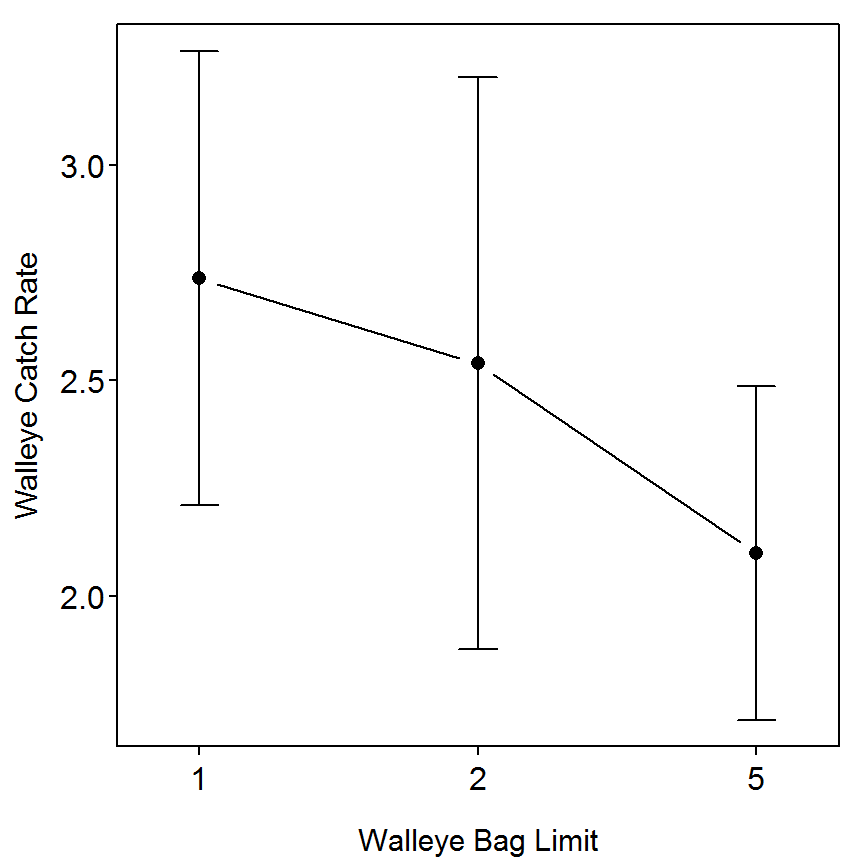### 3.8.5 ANOVA Results II

The same model fits summarized with type-II SS are obtained with Anova() including the type="III" argument. The conclusions from these summaries are identical to the conclusions from above.

> Anova(lm1,type="II")
            Sum Sq      Df F value Pr(>F)
fbag_limit  1.6232  2.0000   2.426 0.1153
Residuals   6.3561 19.0000
Total      21.0000  7.9793               

## 3.9 Example of How to Test Errors (Residuals) for Normality

In an extension to the example in BOX 3.8, the results of the analysis were augmented to examine the normality of residuals.

The analysis below requires the same data and lm1 linear model object fit in BOX 3.8 above.

### 3.9.1 Testing the Normality Assumption

R does not have one function that prints out the four tests of normality as shown in Box 3.9 in the text. However, all four tests of normality shown in Box 3.9 can be accomplished with the following functions,

• ad.test(): performs Anderson-Darling test (ad.test() is from the nortest} package).
• cvm.test()}: performs Cramer-von Mises test (cvm.test()is from thenortest package).
• shapiro.test(): performs Shapiro-Wilk test
• ks.test(): performs Kolmogorov-Smirnov test.

Each of these functions requires the residuals from the linear model fit as the first argument. These residuals are extracted by appending $residuals to the lm object name. > lm1$residuals               # for demonstration purposes only
           1            2            3            4            5            6
-0.526666667 -0.416666667  0.003333333 -0.310000000 -0.290000000 -1.140000000
7            8            9           10           11           12
-0.180000000 -0.320000000 -0.460000000 -0.130000000 -0.110000000 -0.036666667
13           14           15           16           17           18
0.893333333  0.083333333  0.550000000  1.090000000  0.280000000  0.100000000
19           20           21           22
-0.360000000  0.750000000  0.910000000 -0.380000000 
> ad.test(lm1$residuals) Anderson-Darling normality test with lm1$residuals
A = 0.7002, p-value = 0.05793
> cvm.test(lm1$residuals) Cramer-von Mises normality test with lm1$residuals
W = 0.1207, p-value = 0.05387
> shapiro.test(lm1$residuals) Shapiro-Wilk normality test with lm1$residuals
W = 0.9336, p-value = 0.146

The test statistics for the Anderson-Darling and Cramer-von Mises tests are the same as Box 3.9 in the text, but the p-value are slightly different.

The ks.test() function for performing the Kolmogorov-Smirnov test is a more general function that can test whether the residuals follow any possible continuous distribution. To use this function to simply test one set of data for normality you must tell the function, as the second argument to the function, to test against a cumulative normal distribution. In R, the pnorm() function contains the cumulative normal distribution. Thus, a Kolmogorov-Smirnov test of normality is conducted as shown below.

> ks.test(lm1$residuals,"pnorm") One-sample Kolmogorov-Smirnov test with lm1$residuals
D = 0.2538, p-value = 0.09785
alternative hypothesis: two-sided 

The results for the Kolmogorov-Smirnov test are not the same as in Box 3.9 in the text.

The Q-Q normal probability plot is constructed by submitting the residuals from the linear model to qqnorm(). Personally, I also like to see the histogram of residuals constructed by submitting the linear model residuals to hist().

> qqnorm(lm1$residuals,main="")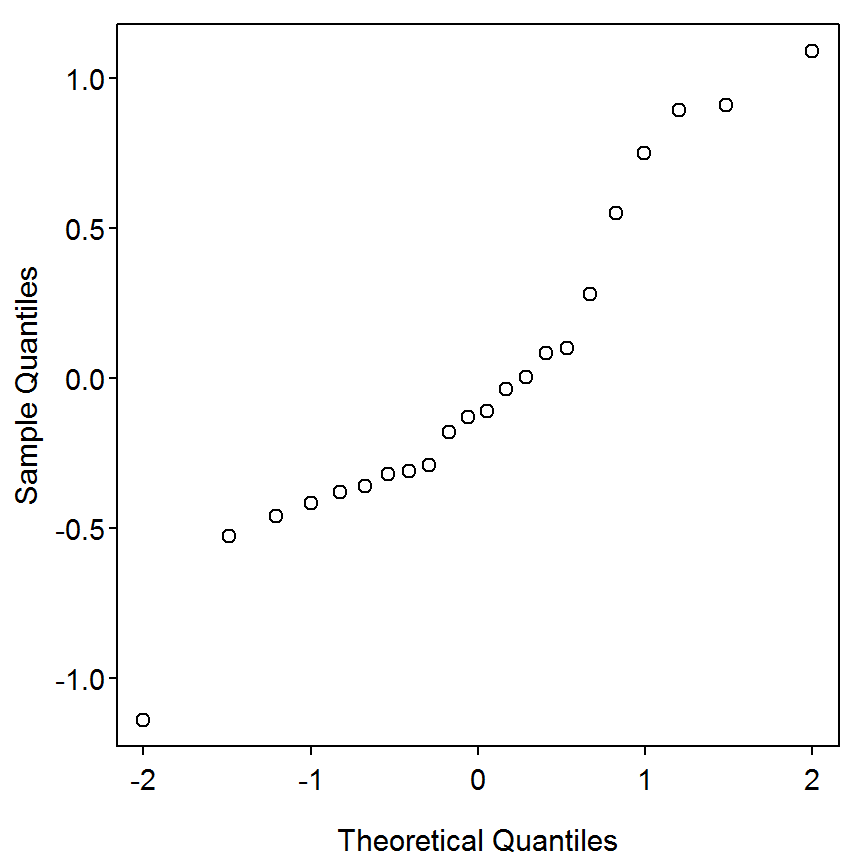> hist(lm1$residuals,main="")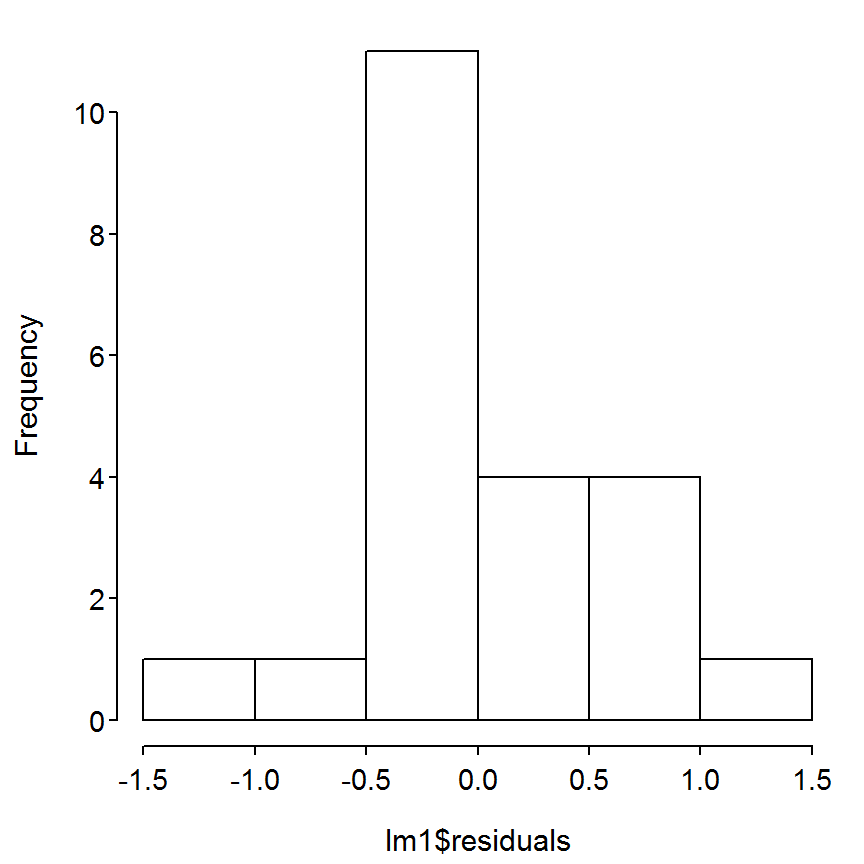### 3.9.2 Testing the Equal Variances Assumption

The authors of Chapter 3 mention two tests (Bartlett’s and Levene’s) for equal variances without showing how to perform these tests. In R, these tests are performed with bartlett.test() and leveneTest(), from the car package, respectively. Both of these functions can take the same linear model formula as their first argument with the data= argument. However, as a matter of simplicity, leveneTest() can also take the lm object as it’s first argument,

> bartlett.test(catch~fbag_limit,data=d8)
Bartlett test of homogeneity of variances with catch by fbag_limit
Bartlett's K-squared = 1.0397, df = 2, p-value = 0.5946
> leveneTest(catch~fbag_limit,data=d8)
      Df F value Pr(>F)
group  2   0.491 0.6196
19               
> leveneTest(lm1)
      Df F value Pr(>F)
group  2   0.491 0.6196
19               

The boxplot of the residuals, constructed by submitting the lm object to residPlot(), from the FSA package, is used to produce a general graphic for visualizing the variability among groups.

> residPlot(lm1)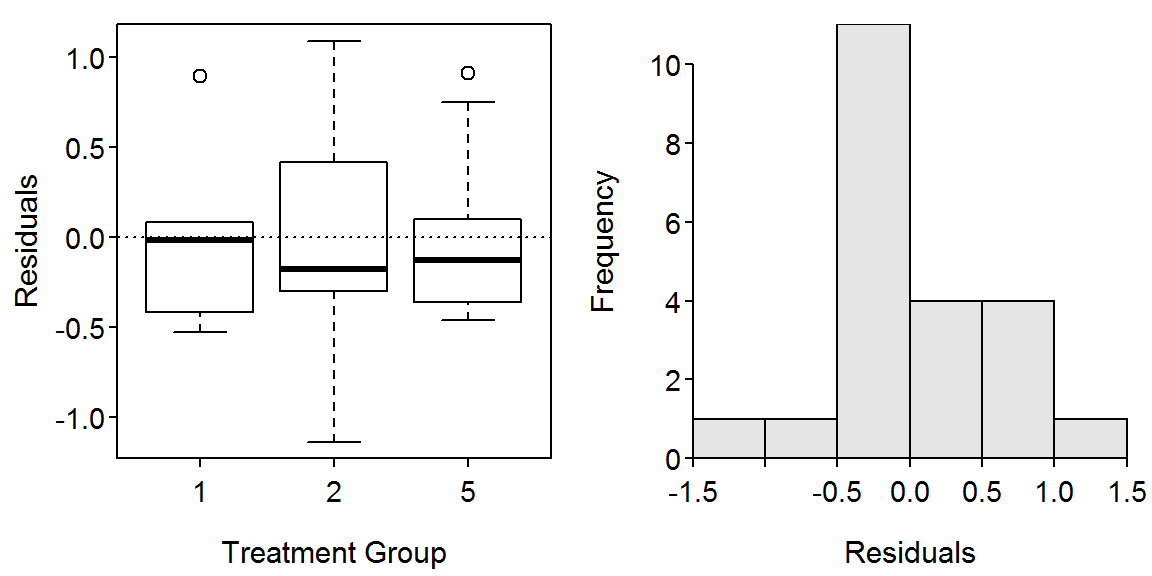### 3.9.3 Outlier Detection

The authors of Chapter 3 mentioned that outliers can be problematic in linear modeling. They mention that individuals with residuals more than three standard deviations from the mean may be considered to be “outliers.” A Studentized residual is essentially a residual that has been standardized to approximately follow a t-distribution. Thus, any individual with an absolute value studentized residual greater than three could be considered an outlier with the author’s suggestion. Studentized residuals for each individual are computed in R by submitting the lm object to studres().

> studres(lm1)
           1            2            3            4            5            6
-0.997346761 -0.781008515  0.006144826 -0.568511579 -0.531238189 -2.374623760
7            8            9           10           11           12
-0.328156405 -0.576418247 -0.836878964 -0.232385953 -0.196550543 -0.067601593
13           14           15           16           17           18
1.786918177  0.153721287  1.028680075  2.240563324  0.512647324  0.178649043
19           20           21           22
-0.650066160  1.410748935  1.758219998 -0.687102631 

As a more objective measure, outlierTest(), from the car package, with the lm object as its only argument, will extract the largest residual and provide a p-value that uses the Bonferroni method to correct for inflated Type-I errors due to multiple comparisons. The null hypothesis for this test is that the individual is NOT an outlier. The result below indicates that the most probable outlier is individual six and it is likely not an outlier (i.e., large Bonferroni p-value).

> outlierTest(lm1)

No Studentized residuals with Bonferonni p < 0.05
Largest |rstudent|:
6 -2.374624           0.028891      0.63559

## 3.10 Example of a Randomized Block Design

In an extension to the analysis of BOXES 3.8 and 3.9, lakes were first blocked into northern and southern Wisconsin lakes, and then treatments were randomly assigned to lakes in each block. A randomized block design should include the blocking factor during the randomization process. The R code for this analysis is similar to a completely randomized design, except that a blocking and an interaction variable are included in the model.

The same data used in BOXES 3.8 and 3.9 are used here.

### 3.10.1 ANOVA Results I

The blocked ANOVA is fit using lm() with a formula where the right-hand-side is the apparent multiplication of the block factor variable and the group factor variable. In R, the apparent multiplication of two factor variables in a linear model formula is a short-hand notation to tell R to include each factor as main effects AND the interaction between the two factors. R denotes the interaction term by separating the main factors with a colon. I prefer to include the blocking variable first in the analysis as the general idea is to remove the variability associated with this variable. It does not, however, make a difference to the results in a complete block design using Type-III SS.

> lm1 <- lm(catch~region*fbag_limit,data=d8)

The type-III SS are extracted by submitting the lm object to Anova() with the type="III" argument. The least-squares means are extracted by submitting the lm object to lsmeans(). As there are two factors and an interaction in this model, R must be told to compute the least-squares means separately by including the factor variable names separately in the second argument’s formula (note that it is likely better to fit a new model without the insignificant interaction term before computing the least-squares means, as noted by the warning).

> Anova(lm1,type="III")
                   Sum Sq     Df  F value    Pr(>F)
(Intercept)       129.935   1.00 660.3029 1.948e-14
region              2.862   1.00  14.5428  0.001529
fbag_limit          1.935   2.00   4.9171  0.021647
region:fbag_limit   0.439   2.00   1.1142  0.352339
Residuals           3.148  16.00
Total              22.000 138.32                   
> lsmeans(lm1,~region)
NOTE: Results may be misleading due to involvement in interactions
 region   lsmean        SE df lower.CL upper.CL
North  2.109444 0.1349830 16 1.823293 2.395596
South  2.844667 0.1376562 16 2.552849 3.136485

Results are averaged over the levels of: fbag_limit
Confidence level used: 0.95 
> lsmeans(lm1,~fbag_limit)
NOTE: Results may be misleading due to involvement in interactions
 fbag_limit   lsmean        SE df lower.CL upper.CL
1          2.736667 0.1810987 16 2.352755 3.120579
2          2.620000 0.1694023 16 2.260883 2.979117
5          2.074500 0.1487878 16 1.759084 2.389916

Results are averaged over the levels of: region
Confidence level used: 0.95 

The fitPlot() function is used to visually observe the mean (with default 95% CIs) catch rates of the different bag limit groups in the different regions. The change.order= argument is used to change which variable is plotted on the x-axis (you may have to make this plot once before deciding which way you prefer it).

> fitPlot(lm1,change.order=TRUE,xlab="Walleye Bag Limit",ylab="Walleye Catch Rate",main="")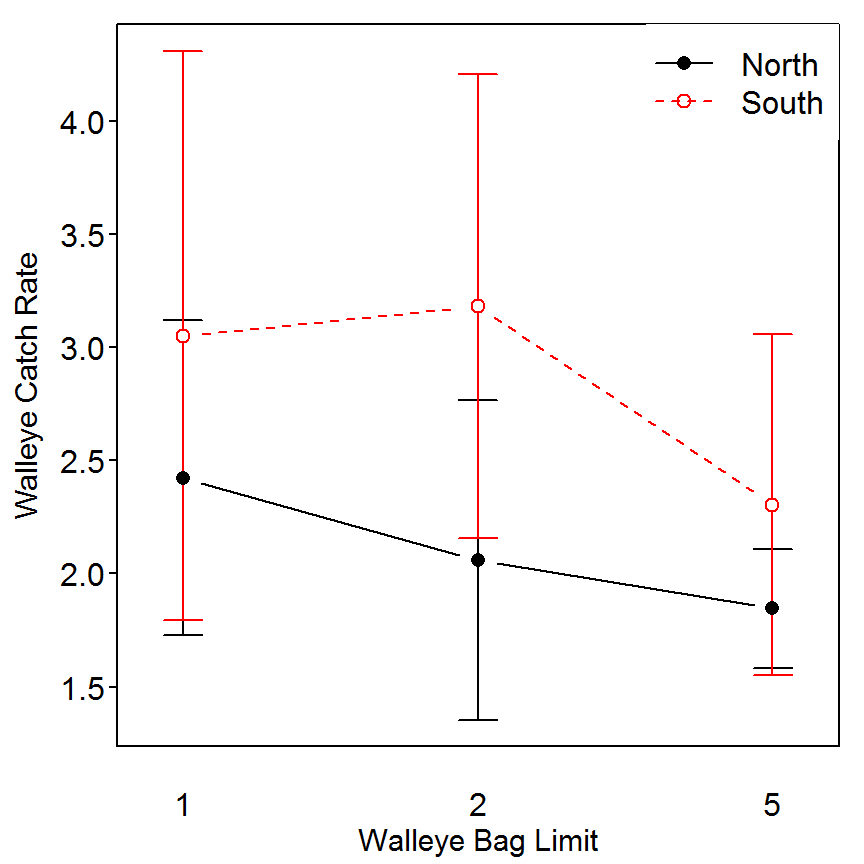As the interaction term is insignificant, means (with 95% CI) plots for the main effects can be obtained with fitPlot() using the which= argument set equal to the name of the factor variable to be shown.

> fitPlot(lm1,which="fbag_limit",xlab="Walleye Bag Limit",ylab="Walleye Catch Rate",main="")> fitPlot(lm1,which="region",xlab="Region",ylab="Walleye Catch Rate",main="")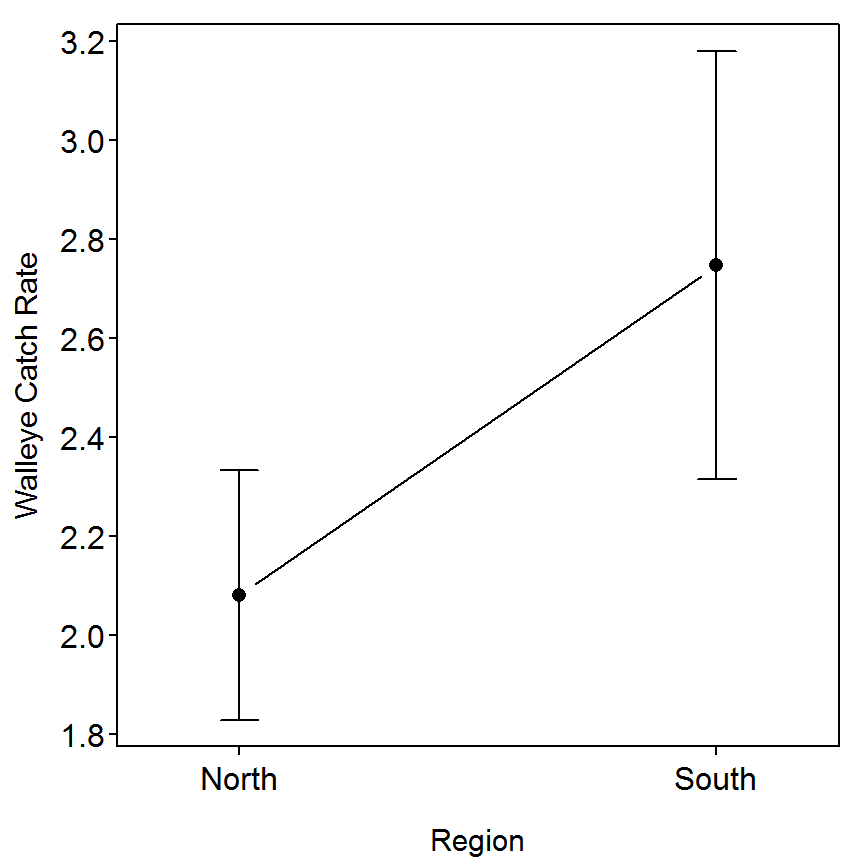Many authors warn against interpretations between the levels of the blocking variable because the blocks were included in the analysis because there was an a priori idea of a difference between the levels and because the levels were generally not randomized. I have presented the block-levels comparisons here because they were presented in Chapter 3.

### 3.10.2 ANOVA Results II

The model fit without the interaction term, followed by the type-III SS ANOVA table, and least-squares means is shown below.

> lm2 <- lm(catch~region+fbag_limit,data=d8)
> Anova(lm2,type="III")
             Sum Sq     Df  F value    Pr(>F)
(Intercept) 129.748   1.00 651.0897 1.386e-15
region        2.769   1.00  13.8958  0.001541
fbag_limit    1.957   2.00   4.9096  0.019877
Residuals     3.587  18.00
Total        22.000 138.06                   
> lsmeans(lm2,~fbag_limit)
 fbag_limit   lsmean        SE df lower.CL upper.CL
1          2.736667 0.1822443 18 2.353786 3.119548
2          2.590978 0.1692787 18 2.235337 2.946620
5          2.060350 0.1491815 18 1.746932 2.373769

Results are averaged over the levels of: region
Confidence level used: 0.95 
> lsmeans(lm2,~region)
 region   lsmean        SE df lower.CL upper.CL
North  2.105818 0.1352208 18 1.821730 2.389907
South  2.819512 0.1366475 18 2.532426 3.106598

Results are averaged over the levels of: fbag_limit
Confidence level used: 0.95 

### 3.10.3 ANOVA Results III

The same model fits summarized with type-II SS are extracted by submitting the lm objects to Anova() with type="II". The conclusions from these summaries are identical to the conclusions from above.

> Anova(lm1,type="II")
                   Sum Sq      Df F value   Pr(>F)
region             2.7691  1.0000 14.0722 0.001743
fbag_limit         1.9567  2.0000  4.9719 0.020926
region:fbag_limit  0.4385  2.0000  1.1142 0.352339
Residuals          3.1485 16.0000
Total             21.0000  8.3129                 
> Anova(lm2,type="II")
            Sum Sq      Df F value   Pr(>F)
region      2.7691  1.0000 13.8958 0.001541
fbag_limit  1.9567  2.0000  4.9096 0.019877
Residuals   3.5870 18.0000
Total      21.0000  8.3129                 

## 3.11 Example of an Analysis of Covariance Design

The goal of this study was to determine how substrate size affected early growth of brook trout (Salvelinus fontinalis) eggs. In a lab experiment, a fisheries scientist placed individual brook trout eggs into containers with different substrates. The investigator also believed that egg diameter would affect early growth, so egg size was measured as a continuous covariate. An analysis of covariance model with egg diameter as the continuous variable and substrate as the categorical treatment variable follows.

### 3.11.1 Preparing Data

The box3_11.txt is read and the structure of the data frame is observed below.

> d11 <- read.table("data/box3_11.txt",header=TRUE)
> str(d11)
'data.frame':   29 obs. of  4 variables:
$id : int 1 2 3 4 5 6 7 8 9 10 ...$ substrate   : Factor w/ 3 levels "Cobble","Gravel",..: 1 1 1 1 1 1 1 1 1 1 ..
$egg_diameter: num 8.3 8.5 11.2 10.7 9.6 11.8 9.6 8.9 11.2 8.9 ...$ growth      : num  20 23.5 24.7 29.5 24.3 31.7 22.1 19 17.3 23.3 ...

### 3.11.2 ANCOVA Results I

The ANCOVA is fit with lm() with a formula where the right-hand-side is the apparent multiplication of the quantitative covariate variable and the group factor variable (the meaning of this apparent multiplication is described in BOX 3.10). The type-III SS are obtained by submitting the lm object to Anova() with type="III".

> lm1 <- lm(growth~egg_diameter*substrate,data=d11)
> Anova(lm1,type="III")
                       Sum Sq     Df F value    Pr(>F)
(Intercept)            138.85    1.0  11.944 0.0021464
egg_diameter           557.07    1.0  47.917 4.674e-07
substrate              266.12    2.0  11.445 0.0003548
egg_diameter:substrate 311.46    2.0  13.395 0.0001389
Residuals              267.39   23.0
Total                   29.00 1540.9                  

**The right-hand-side of the lm() formula for an ANCOVA should be of the form quantitative*factor, where quantitative represents the quantitative covariate variable and factor represents the categorical group factor variable.**

The fitPlot() function is used to visually observe the regression fit between final length (i.e., growth) and egg diameter for each substrate type.

> fitPlot(lm1,xlab="Egg Diameter (mm)",ylab="Final length (mm)",legend="topleft",main="")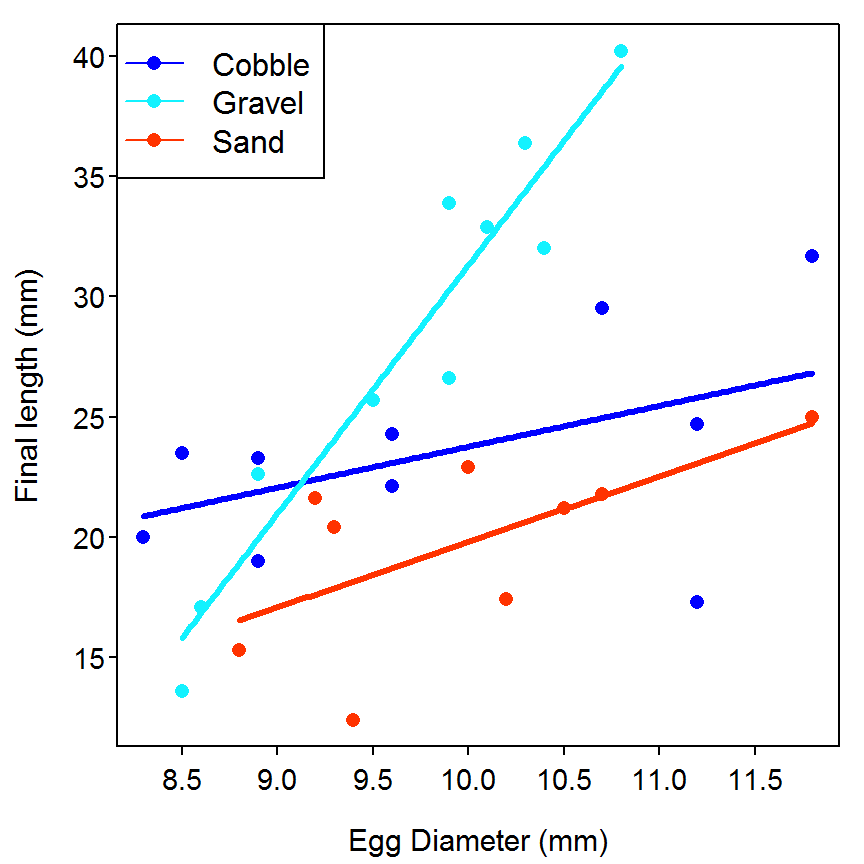### 3.11.3 ANCOVA Results II

The anova table of type II SS is extracted by submitting the lm object to Anova() with type="II". The p-value for the interaction ($$p=0.0001$$) is the same as that shown for the type-III SS because this was the last variable added to the model. Nevertheless, the interaction term is significant indicating a different slope between final length (i.e., growth) and egg diameter among the three substrate types.

> Anova(lm1,type="II")
                       Sum Sq   Df F value    Pr(>F)
egg_diameter           383.07    1  32.951 7.585e-06
substrate              420.10    2  18.068 1.921e-05
egg_diameter:substrate 311.46    2  13.395 0.0001389
Residuals              267.39   23
Total                   28.00 1382                  

## 3.12 Example of a Mixed-Model Design

The goal of this study was to determine the effect of herbicide treatment on the abundance of age-0 bluegill (Lepomis macrochirus) in lakes. In theory, treatment with herbicide will create greater access to food resources, so abundance of age-0 bluegill should increase. Funds were available for treating and sampling only four lakes each year, along with sampling an equivalent number of untreated control lakes. To increase the sample size available for the experiment, the fisheries scientists treated lakes over four years but were concerned that year-to-year variation in weather could obscure the real effect of treatment.

### 3.12.1 Preparing Data

The box3_12.txt is read and the structure of the data frame is observed below. The year and lakeID (though the latter is not used in this Box) are converted to group factor variables with factor().

> d12 <- read.table("data/box3_12.txt",header=TRUE)
> str(d12)
'data.frame':   32 obs. of  4 variables:
$year : int 2001 2001 2001 2001 2001 2001 2001 2001 2002 2002 ...$ herbicide: Factor w/ 2 levels "Control","Treatment": 2 2 2 2 1 1 1 1 2 2 ...
$lakeID : int 988 116 375 17 592 677 850 566 814 397 ...$ bluegill : int  86 100 163 135 62 69 56 50 172 200 ...
> d12$year <- factor(d12$year)
> d12$lakeID <- factor(d12$lakeID)
> str(d12)
'data.frame':   32 obs. of  4 variables:
$year : Factor w/ 4 levels "2001","2002",..: 1 1 1 1 1 1 1 1 2 2 ...$ herbicide: Factor w/ 2 levels "Control","Treatment": 2 2 2 2 1 1 1 1 2 2 ...
$lakeID : Factor w/ 32 levels "17","35","76",..: 31 6 12 1 22 24 28 20 27 ..$ bluegill : int  86 100 163 135 62 69 56 50 172 200 ...

### 3.12.2 Quick Exploration

The tapply() function is used to create a two-way table with the results of a function making up the cells of the table. This function requires the vector of numerical data to be summarized as the first argument, the group factor variables that form the rows and columns in a list as the second arguments, and the function that will summarize the data in the FUN= argument. For example, tables of the means and standard deviations for each year and herbicide treatment combination are constructed below.

> round(tapply(d12$bluegill,list(d12$herbicide,d12$year), FUN=mean),1) # round for display only  2001 2002 2003 2004 Control 59.2 85.5 106.5 63.5 Treatment 121.0 182.2 129.5 69.0 > round(tapply(d12$bluegill,list(d12$herbicide,d12$year), FUN=sd),1)
          2001 2002 2003 2004
Control    8.1 45.2 67.7 52.6
Treatment 34.8 24.1 16.0 10.2

A boxplot of each year and herbicide treatment combination is constructed with bwplot() from the lattice package. This function requires a formula of the form +response~factor1|factor2 where factor2 to the right of the | represents the group factor variable that dictates new “panels” for the plot.

> bwplot(bluegill~herbicide|year,data=d12)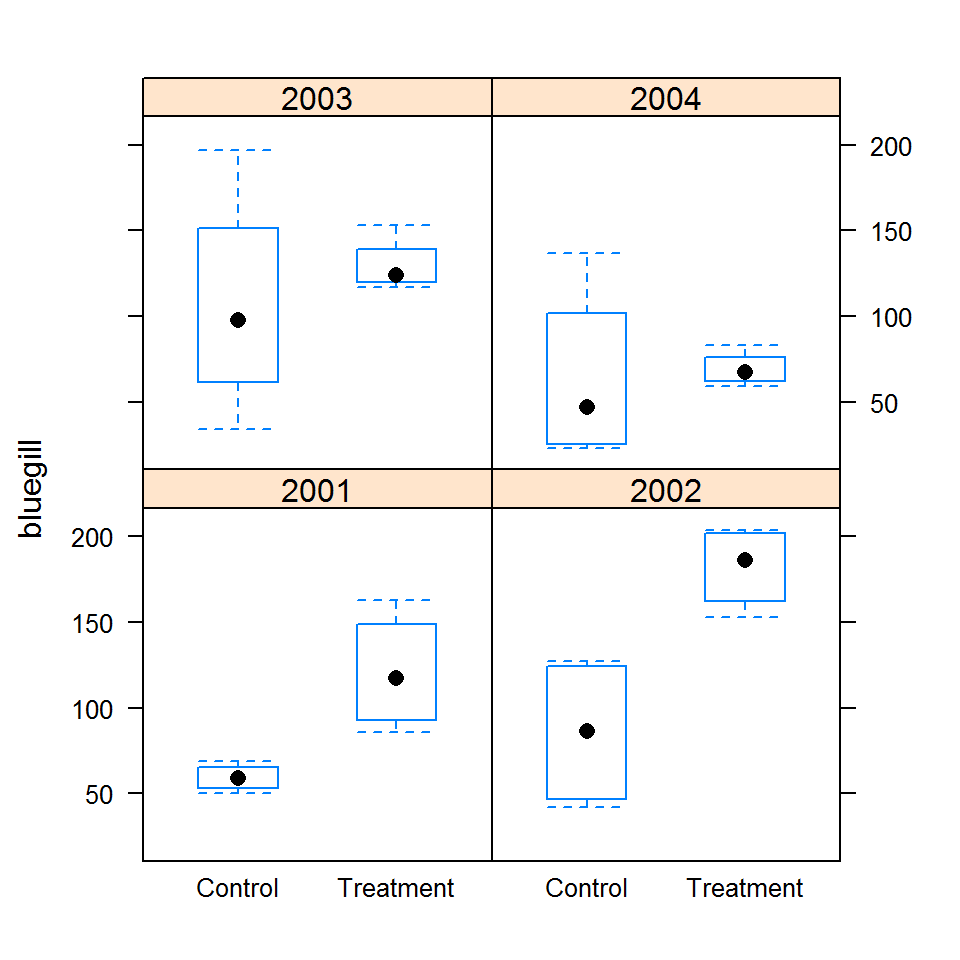The summary statistics and boxplot indicate that the relationship of the means between the herbicide treatments varies fairly dramatically from year-to-year. In addition, the variability varies dramatically between years and herbicide treatments without an apparent pattern.

### 3.12.3 Fitting the Mixed-Effects Model in the Box

Mixed-effects models are fit in R with lmer() from the lme4 package. The formula notation in lmer() has two parts that correspond to the fixed and random components of the model. The random effect term must be contained in quotes so that it is parsed correctly; thus, the fixed effects are “left out of parentheses.” In the example below the fixed effects portion of the formula is contained in 1+herbicide. In this case the 1 indicates that an overall intercept term should be fit (e.g., if a 0 had been used then estimates for each level in herbicide rather than a difference from the “first” level would have been computed). Also, in the example below, the random effects portion of the formula is contained in (1|year). The 1 in this portion of the formula indicates the “intercept” and the |year portion indicates that a different intercept will be fit for each year. Finally, lmer() takes a data= argument and the optional REML=TRUE argument if restrictive maximum likelihood methods are to be used (set this to FALSE to use straight maximum likelihood methods (REML=TRUE is the default and does not need to be specified. I specified it in this example so as to explicitly note that that is what the SAS code in Box 3.12 in the text used).

> lme1 <- lmer(bluegill~1+herbicide+(1|year),data=d12,REML=TRUE)

The random components in the mixed effects model must be contained in parentheses within the formula of lmer().

Several extractor functions are used to extract various information from this model fit. Before showing these results it should be noted that there is considerable debate over exactly how to construct default hypothesis tests in mixed-effects models (primarily surrounding the philosophy of identifying the correct degrees-of-freedom). As such, the author of lme4 has opted to not print p-values by default in the R output. For this reason, it is not possible to reconstruct the exact output shown in Box 3.12.

Default p-values are not reported in the R output for mixed-effects models fit with lmer() from lme4. This was a conscious decision by the package creator because of debate about the correct degrees-of-freedom to use in these tests.

Basic information regarding the model fit is extracted by submitting the saved lmer object to summary() (alternatively, you can use display() from the arm package). The AIC, BIC, and log likelihood are printed near the top of the output and closely resemble that shown on the bottom of page 101 (with the exception that R outputs the log likelihood and SAS outputs -2 times the log likelihood). The variance estimates are printed under the “Random Effects” heading. The residual variance is 1661 and the variance among years is 689. These are identical to what is shown near the bottom of page 101. Note that the “Std. Dev” column in the R output is simply the square root of the “Variance” column and NOT the standard errors as shown in Box 3.12 in the text.

> summary(lme1)
Linear mixed model fit by REML ['lmerMod']
Formula: bluegill ~ 1 + herbicide + (1 | year)
Data: d12

REML criterion at convergence: 318.9

Scaled residuals:
Min      1Q  Median      3Q     Max
-1.5003 -0.5991 -0.2478  0.4606  2.6027

Random effects:
Groups   Name        Variance Std.Dev.
year     (Intercept)  689.4   26.26
Residual             1660.6   40.75
Number of obs: 32, groups:  year, 4

Fixed effects:
Estimate Std. Error t value
(Intercept)  102.062     14.975   6.815
herbicide1   -23.375      7.204  -3.245

Correlation of Fixed Effects:
(Intr)
herbicide1 0.000 

The conditional means for the random effects shown on page 102 are extracted with ranef(). Standard errors for each of these terms are found with se.ranef() from the arm package. Tests for fixed effects shown in the middle of page 102 are extracted with anova().

> ranef(lme1)
$year (Intercept) 2001 -9.175087 2002 24.450885 2003 12.249461 2004 -27.525260 > se.ranef(lme1) $year
(Intercept)
2001    12.63102
2002    12.63102
2003    12.63102
2004    12.63102
> anova(lme1)
          Df Sum Sq Mean Sq F value
herbicide  1  17485   17485  10.529
Total      1  17485                

I do not know of a specific method for computing the least-squares means from a mixed-effects model. However, if one modifies the model above by fitting a term for each level of the herbicide variable rather than fitting an overall intercept term, then the treatment coefficients are found and they match the least-squares means shown in Box 3.12 in the text. This is illustrated in the fixed-effects portion of the output below.

> lme1a <- lmer(bluegill~0+herbicide+(1|year),data=d12,REML=TRUE)
> smry1a <- summary(lme1a)
> coef(smry1a)
                   Estimate Std. Error  t value
herbicideControl    78.6875   16.61779 4.735137
herbicideTreatment 125.4375   16.61779 7.548387

An alternative to the fixed-effects test is to use a likelihood ratio test to compare the full model with the fixed herbicide term and the model without that term. This likelihood ratio test can be constructed by fitting the two models (note that the model with the fixed herbicide term was fit above and the model without this term is fit below) and then submitting the two model objects to lrt() with the more complex model in com=. Both the p-value ($$p=0.0001$$) and lower AIC/BIC values indicate that the more complex model with the herbicide term is needed. This further implies that there is a difference among the herbicide treatments.

> lme2 <- lmer(bluegill~1+(1|year),data=d12,REML=TRUE)  # fit without fixed term
> lrt(lme2,com=lme1)                                    # perform LRT comparison
Model 1: bluegill ~ 1 + (1 | year)
Model A: bluegill ~ 1 + herbicide + (1 | year)

DfO   logLikO DfA   logLikA Df    logLik  Chisq Pr(>Chisq)
1vA  30 -166.9477  29 -159.4535  1   -7.4942 14.989  0.0001082

### 3.12.4 Predicted Values

Predicted values for each year and herbicide treatment combination are constructed in a fairly manual way in the code below. First, the fixed effects and random effects for year from the model where an overall intercept was not estimated are saved to objects. The fixed effects for the “control” and “herbicide” treatments are then added to the random year effects separately and then combined back together to make a nice table for presentation.

> ( fe1a <- fixef(lme1a) )
  herbicideControl herbicideTreatment
78.6875           125.4375 
> ( re1a <- ranef(lme1a)$year )  (Intercept) 2001 -9.175086 2002 24.450885 2003 12.249461 2004 -27.525259 > pred.C <- fe1a+re1a > pred.H <- fe1a+re1a > pred <- t(cbind(pred.C,pred.H)) > colnames(pred) <- rownames(re1a) > rownames(pred) <- c("Control","Herbicide") > round(pred,1) # rounded for display purposes only.  2001 2002 2003 2004 Control 69.5 103.1 90.9 51.2 Herbicide 116.3 149.9 137.7 97.9 These predictions can be compared to sample means computed above to note that the predictions are off a good deal in some year treatment combinations. > round(tapply(d12$bluegill,list(d12$herbicide,d12$year),FUN=mean)-pred,1)
           2001  2002 2003  2004
Control   -10.3 -17.6 15.6  12.3
Treatment   4.7  32.4 -8.2 -28.9

### 3.12.5 P-values with Markov Chain Monte Carlo Simulation

Markov chain Monte Carlo (MCMC) simulation methods can be used to construct approximate confidence intervals and p-values for testing that a parameter is equal to zero for the fixed and random effect parameters in the mixed effect model. The pvals.fnc() function, from the langaugeR package, performs the MCMC simulations for the results in an lmer object. This function only requires the saved lmer object as the first argument. However, the number of MCMC simulations is set with nsims= and the result of each simulation is returned by including the withMCMC=TRUE argument. The results should be saved to an object where the results for the fixed and random effects are then extracted by appending $fixed and $random to the saved object name.

> ### THIS NEEDS TO BE UPDATED
> p1a <- pvals.fnc(lme1a,nsim=1000,withMCMC=TRUE)
> p1a$fixed > p1a$random

Density curves for the MCMC results for each of the parameter estimates are shown below (the “complex” code basically creates a function that stacks the MCMC results and then plots them using densityplot() from the lattice package.

> mcmcM <- as.matrix(p1a$mcmc) > m <- data.frame(Value=mcmcM[,1],Predictor=rep(colnames(mcmcM),nrow(mcmcM))) > for (i in 2:ncol(mcmcM)) { mtmp <- data.frame(Value=mcmcM[,i],Predictor=rep(colnames(mcmcM)[i],nrow(mcmcM))) m <- rbind(m, mtmp) } > densityplot(~Value|Predictor,data=m,scales=list(relation="free"), par.strip.text=list(cex=0.75),xlab="Posterior Values",ylab="Density",pch=".") ## 3.13 Example of a Factorial Design The goal of this study was to determine how size and stocking location of fingerling Chinook salmon (Oncorhynchus tshawytscha) affected survival and subsequent return to the Snake River. Bugert and Mendel (1997) used a 2x2 factorial design in which size (subyearling versus yearling) and location of release (on-station versus off-station) were compared to see how these factors affected survival. For this example, we have included only years when all treatment combinations were implemented. ### 3.13.1 Preparing Data The box3_13.txt is read and the structure of the data frame is observed below. As noted in Box 3.13, the survival variable should be transformed because values expressed as proportions (or percentages) rarely meet the normality or equal variances assumptions of ANOVA. The typical transformations for variables expressed as proportions is to use the arc-sine (i.e., inverse sine) square-root function. The authors of Box 3.13 used only an arc-sine transformation. In this vignette, I will use the arc-sine transformation to demonstrate equivalence of R and SAS output and then use the arc-sine square root transformation to demonstrate what I see as the “proper” methodology. The survival variable is transformed to the arc-sine of survival (and called arcsurv) with asin(). The arc-sine square root of survival (and called arcsrsurv) is computed similarly but includes the use of sqrt(). > d13 <- read.table("data/box3_13.txt",header=TRUE) > str(d13) 'data.frame': 16 obs. of 4 variables:$ year    : int  1987 1987 1987 1987 1988 1988 1988 1988 1989 1989 ...
$size : Factor w/ 2 levels "Sub","Yearling": 1 1 2 2 1 1 2 2 1 1 ...$ release : Factor w/ 2 levels "Off","On": 2 1 2 1 2 1 2 1 2 1 ...
$survival: num 0.058 0.155 0.406 0.319 0.058 ... > d13$arcsurv <- asin(d13$survival/100) > d13$arcsrsurv <- asin(sqrt(d13$survival/100)) > str(d13) 'data.frame': 16 obs. of 6 variables:$ year     : int  1987 1987 1987 1987 1988 1988 1988 1988 1989 1989 ...
$size : Factor w/ 2 levels "Sub","Yearling": 1 1 2 2 1 1 2 2 1 1 ...$ release  : Factor w/ 2 levels "Off","On": 2 1 2 1 2 1 2 1 2 1 ...
$survival : num 0.058 0.155 0.406 0.319 0.058 ...$ arcsurv  : num  0.00058 0.00155 0.00406 0.00319 0.00058 ...
$arcsrsurv: num 0.0241 0.0394 0.0638 0.0565 0.0241 ... ### 3.13.2 ANOVA Results I The ANOVA, using the arc-sine transformed survival variable, is fit with lm() using a formula where the right-hand-side is the apparent multiplication of the two group factor variables (the meaning of this apparent multiplication is described in BOX 3.10). The type-III SS are obtained by submitting the lm object to Anova() with type="III". > lm1 <- lm(arcsurv~size*release,data=d13) > Anova(lm1,type="III")  Sum Sq Df F value Pr(>F) (Intercept) 0.0003 1.0000000 6.9597 0.02165 size 0.0002 1.0000000 5.4820 0.03729 release 0.0001 1.0000000 1.9489 0.18800 size:release 0.0001 1.0000000 1.8770 0.19577 Residuals 0.0005 12.0000000 Total 16.0000 0.0012081  The least-squares means are extracted by submitting the lm object to lsmeans(). As there are two factors in this model, R must be told to compute the least-squares means separately by including the factor variable names separately in the right-hand-side of the second argument. In addition, if you the interaction term is supplied to the second argument then the least-squares means for all possible groups will be computed. > lsmeans(lm1,~size) NOTE: Results may be misleading due to involvement in interactions  size lsmean SE df lower.CL upper.CL Sub 0.0004850001 0.002311295 12 -0.004550879 0.005520879 Yearling 0.0081381526 0.002311295 12 0.003102273 0.013174032 Results are averaged over the levels of: release Confidence level used: 0.95  > lsmeans(lm1,~release) NOTE: Results may be misleading due to involvement in interactions  release lsmean SE df lower.CL upper.CL Off 0.006593146 0.002311295 12 0.001557267 0.011629025 On 0.002030007 0.002311295 12 -0.003005873 0.007065886 Results are averaged over the levels of: size Confidence level used: 0.95  > lsmeans(lm1,~size*release)  size release lsmean SE df lower.CL upper.CL Sub Off 0.0005275002 0.003268665 12 -0.006594309 0.007649309 Yearling Off 0.0126587916 0.003268665 12 0.005536983 0.019780600 Sub On 0.0004425000 0.003268665 12 -0.006679309 0.007564309 Yearling On 0.0036175136 0.003268665 12 -0.003504295 0.010739322 Confidence level used: 0.95  ### 3.13.3 ANOVA Results II The ANOVA using the arc-sine square-root transformed survival variable is fit similarly. There does not appear to be an interaction between the two factors ($$p=0.1725$$); thus, the main effects can be interpreted. There does not appear to be a difference in the mean arc-sine square root of survival between the two release sites ($$p=0.2007$$). However, the mean arc-sine square root of survival does appear to differ significantly between the two sizes of released fish ($$p=0.0024$$). These are the same qualitative results as was obtained with the arc-sine transformation used in Box 3.13 of the AIFFD book. > lm2 <- lm(arcsrsurv~size*release,data=d13) > Anova(lm2,type="III")  Sum Sq Df F value Pr(>F) (Intercept) 0.0394 1.000000 40.3196 3.666e-05 size 0.0144 1.000000 14.7029 0.002376 release 0.0018 1.000000 1.8329 0.200730 size:release 0.0021 1.000000 2.1046 0.172491 Residuals 0.0117 12.000000 Total 16.0000 0.069388  The fitPlot() function is used to construct an interaction plot where the simultaneous effects of the two factors on the arc-sine square root of survival can be visualized. > fitPlot(lm2,xlab="Release Size",ylab="Arcsine Square Root of Survival",legend="topleft",main="")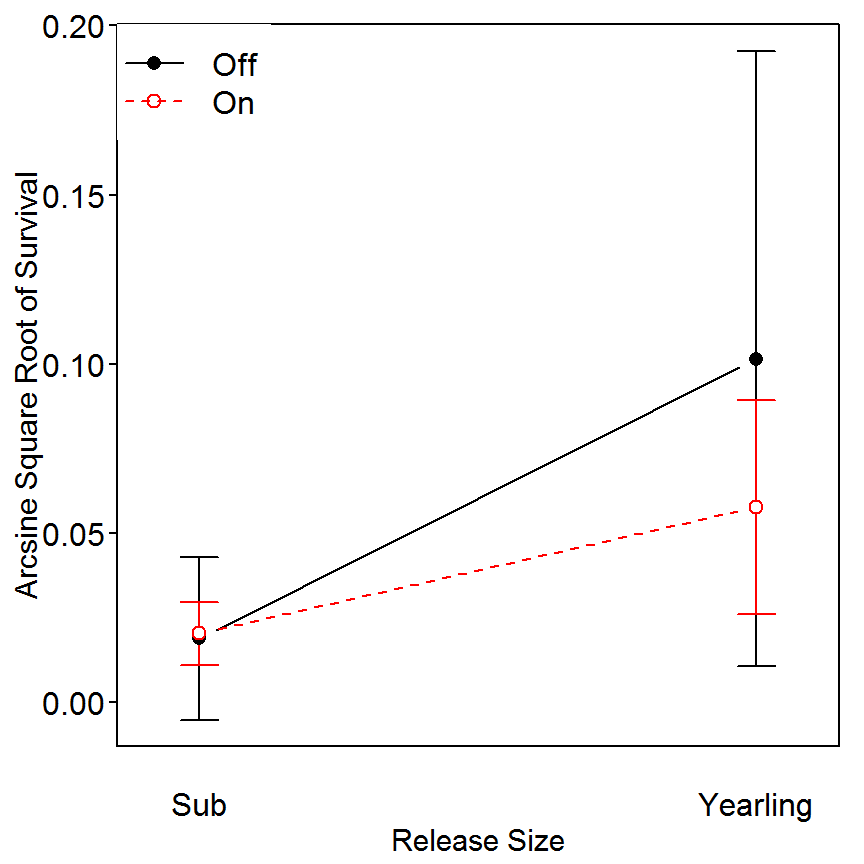However, because the interaction was insignificant, you can also look at the main-effect means plots using fitPlot() with which= set equal to the group factor variables of interest (note that the ylim= arguments were used to control the range of y-axis so as to allow for a better comparison of the relative effects of the two main effects). > fitPlot(lm2,which="size",xlab="Release Size",ylab="Arcsine Square Root of Survival",legend="topleft",main="",ylim=c(0.01,0.12))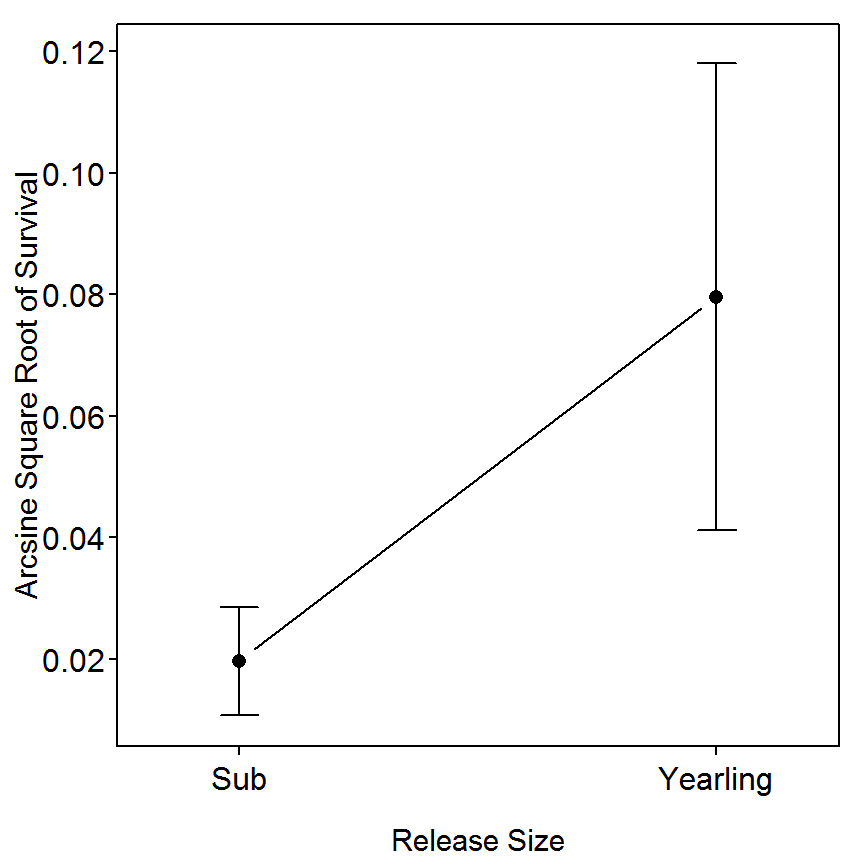> fitPlot(lm2,which="release",xlab="Release Site",ylab="Arcsine Square Root of Survival",legend="topleft",main="",ylim=c(0.01,0.12))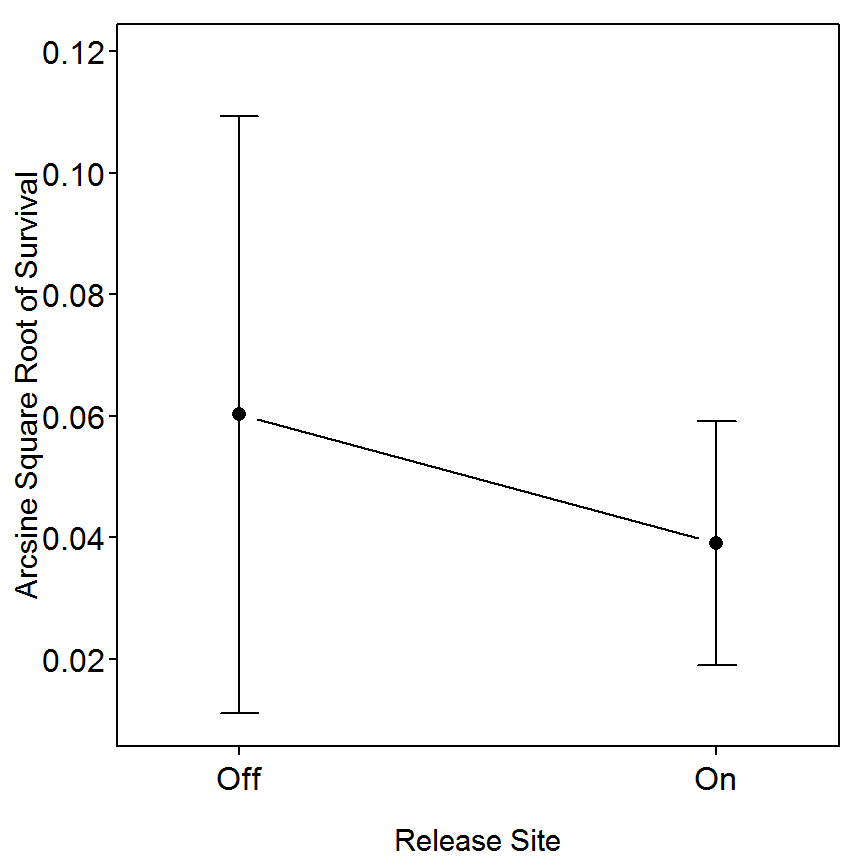### 3.13.4 ANOVA Results III The same model fits summarized with type-II SS are shown below. The conclusions from these summaries are identical to the conclusions from above. > Anova(lm1,type="II")  Sum Sq Df F value Pr(>F) size 0.0002 1.0000e+00 5.4820 0.03729 release 0.0001 1.0000e+00 1.9489 0.18800 size:release 0.0001 1.0000e+00 1.8770 0.19577 Residuals 0.0005 1.2000e+01 Total 15.0000 9.1063e-04  > Anova(lm2,type="II")  Sum Sq Df F value Pr(>F) size 0.0144 1.000000 14.7029 0.002376 release 0.0018 1.000000 1.8329 0.200730 size:release 0.0021 1.000000 2.1046 0.172491 Residuals 0.0117 12.000000 Total 15.0000 0.029962  ## 3.14 Example of a Nested Design For the example in Box 3.12, where the effect of herbicide treatment on age-0 bluegill (Lepomis macrochirus) density was investigated, we may also be interested in how herbicide treatment affects mean length of age-0 bluegill at the end of the growing season (for this example, assume that length of individual bluegill from each lake in the study was measured). In a nested design, the primary experimental unit is a lake, so each bluegill is not an independent replicate but rather is a subsample from the lake. For brevity, only the lakes sampled in 2001 from Box 3.12 are used in this example. ### 3.14.1 Preparing Data The box3_14.txt is read, the lake variable is converted to a factor, and the structure of the data frame is observed below. > d14 <- read.table("data/box3_14.txt",header=TRUE) > d14$lake <- factor(d14$lake) > str(d14) 'data.frame': 80 obs. of 3 variables:$ herbicide: Factor w/ 2 levels "Control","Treatment": 2 2 2 2 2 2 2 2 2 2 ...
$lake : Factor w/ 8 levels "17","116","375",..: 8 8 8 8 8 8 8 8 8 8 ...$ length   : int  103 90 98 90 96 88 97 100 89 108 ...

### 3.14.2 Quick Exploration

A boxplot of fish lengths by each herbicide and lake combination is constructed with bwplot() from the lattice package. This function requires a formula as the first argument. In this example, the formula will be of the form response~(factor1:factor2) so that the x-axis will be labeled with the herbicide treatment and the lake number. Corresponding summary statistics are computed with Summarize() from the FSA package. This function requires the same type of formula as used in bwplot() (note that digits= controls the number of outputted digits). Both of these summaries suggest that the mean length is generally greater in the “treatment” lakes and that lake 677 has a considerably lower variability in length.

> bwplot(length~(herbicide:lake),data=d14)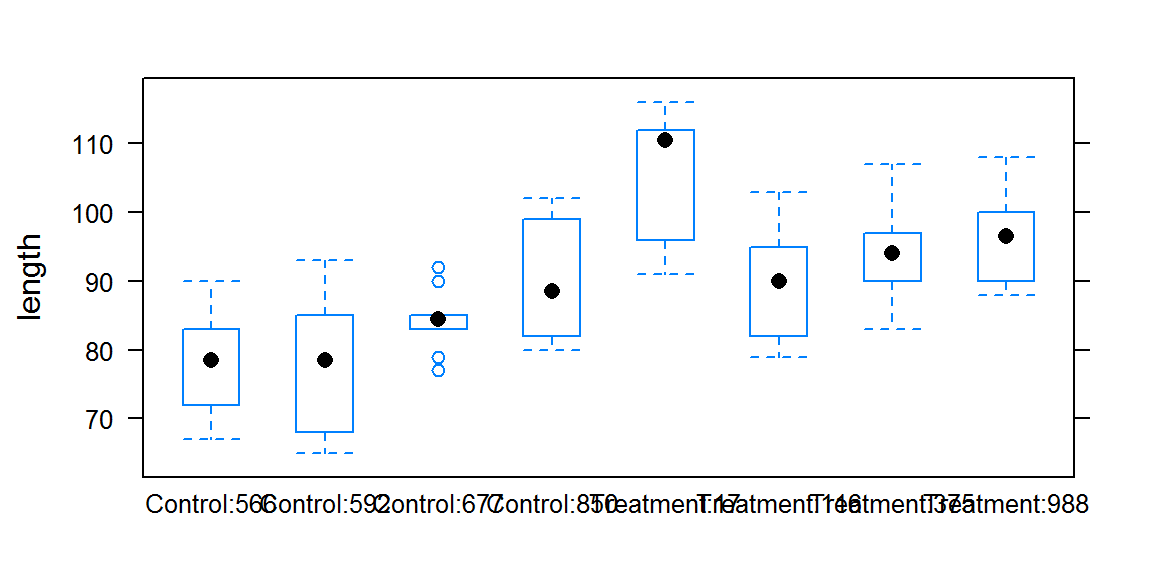> Summarize(length~(lake:herbicide),data=d14,digits=1)
  lake herbicide  n nvalid  mean   sd min   Q1 median    Q3 max percZero
1  566   Control 10     10  77.8  7.6  67 72.8   78.5  82.0  90        0
2  592   Control 10     10  77.2 10.0  65 68.5   78.5  83.8  93        0
3  677   Control 10     10  84.3  4.4  77 83.0   84.5  85.0  92        0
4  850   Control 10     10  89.9  8.0  80 83.0   88.5  97.5 102        0
5   17 Treatment 10     10 106.6  9.3  91 99.2  110.5 111.8 116        0
6  116 Treatment 10     10  89.8  8.3  79 82.5   90.0  94.8 103        0
7  375 Treatment 10     10  94.1  7.2  83 90.8   94.0  96.2 107        0
8  988 Treatment 10     10  95.9  6.7  88 90.0   96.5  99.5 108        0

### 3.14.3 Fitting Mixed Effects Model

Mixed-effects models are fit in R with lmer() (use of lmer() was defined more thoroughly in BOX 3.12). In the example below, the fixed effects portion of the formula is contained in 1+herbicide. In this case the 1 indicates that an overall intercept term should be fit (if a “0” had been used then estimates for each level in herbicide rather than a difference from the “first” level would have been computed). Also, in the example below, the random effects portion of the formula is contained in (1|lake:herbicide). The 1 in this portion of the formula indicates the “intercept” and the |lake:herbicide portion indicates that a different intercept will be fit for each lake nested within the herbicide treatments.

> lme1 <- lmer(length~1+herbicide+(1|lake:herbicide),data=d14,REML=TRUE)

Basic information is extracted from the model fit with summary(). The AIC, BIC, and log likelihood are printed near the top of the output and closely resemble that shown on the middle of page 111 (with the exception that R outputs the log likelihood and SAS outputs -2 times the log likelihood). The variance estimates are printed under the “Random Effects” heading. The residual variance is 61.5 and the variance among lakes is 37.4. These are identical to what is shown near the bottom of page 111. Note that the “Std. Dev” column in the R output is simply the square root of the “Variance” column and NOT the standard errors as shown in the Box. The test for fixed effects shown near the bottom of page 111 is extracted with anova().

> summary(lme1)
Linear mixed model fit by REML ['lmerMod']
Formula: length ~ 1 + herbicide + (1 | lake:herbicide)
Data: d14

REML criterion at convergence: 563.1

Scaled residuals:
Min       1Q   Median       3Q      Max
-1.80896 -0.86422  0.01616  0.74135  1.92280

Random effects:
Groups         Name        Variance Std.Dev.
lake:herbicide (Intercept) 37.35    6.111
Residual                   61.50    7.842
Number of obs: 80, groups:  lake:herbicide, 8

Fixed effects:
Estimate Std. Error t value
(Intercept)   89.450      2.332   38.36
herbicide1    -7.150      2.332   -3.07

Correlation of Fixed Effects:
(Intr)
herbicide1 0.000 
> anova(lme1)
          Df Sum Sq Mean Sq F value
herbicide  1 578.21  578.21  9.4018
Total      1 578.21                

An alternative to the fixed-effects test is to use a likelihood ratio test to compare the full model with the fixed herbicide term and the model without that term (as was described in Box 3.12). Both the p-value ($$p=0.0015$$) and lower AIC/BIC values indicate that the more complex model with the herbicide term is needed. This further implies that there is a difference among the herbicide treatments.

> lme2 <- lmer(length~1+(1|lake:herbicide),data=d14,REML=TRUE) # fit without fixed term
> lrt(lme2,com=lme1)                                           # perform LRT comparison
Model 1: length ~ 1 + (1 | lake:herbicide)
Model A: length ~ 1 + herbicide + (1 | lake:herbicide)

DfO   logLikO DfA   logLikA Df    logLik  Chisq Pr(>Chisq)
1vA  78 -286.5962  77 -281.5706  1   -5.0256 10.051   0.001522

I do not know of a specific method for computing the least-squares means from a mixed-effects model. However, if one modifies the model above by fitting a term for each level of the herbicide variable rather than fitting an overall intercept term then the treatment coefficients can be found and they match the least-squares means shown at the bottom of the Box. For example, inspect the fixed-effects portion of the output below.

> lme1a <- lmer(length~0+herbicide+(1|lake:herbicide),data=d14,REML=TRUE)
> smry1a <- summary(lme1a)
> coef(smry1a)
                   Estimate Std. Error  t value
herbicideControl       82.3   3.297726 24.95659
herbicideTreatment     96.6   3.297726 29.29291

### 3.14.4 Examining Random Effects

The conditional means for the random effects are extracted with ranef(). Standard errors for each of these terms are found with se.ranef() from the arm package.

> ranef(lme1)
$lake:herbicide (Intercept) 17:Treatment 8.5862069 116:Treatment -5.8386207 375:Treatment -2.1465517 566:Control -3.8637931 592:Control -4.3789655 677:Control 1.7172414 850:Control 6.5255172 988:Treatment -0.6010345 > se.ranef(lme1) $lake:herbicide
(Intercept)
17:Treatment     2.297938
116:Treatment    2.297938
375:Treatment    2.297938
566:Control      2.297938
592:Control      2.297938
677:Control      2.297938
850:Control      2.297938
988:Treatment    2.297938

### 3.14.5 P-values with Markov Chain Monte Carlo Simulation

Markov chain Monte Carlo (MCMC) simulation methods are used to construct approximate confidence intervals and p-values for testing that a parameter is equal to zero for the fixed and random effect parameters in the mixed effect model. Use of the functions below was described in detail in BOX 3.12.

> #### THIS NEEDS TO BE UPDATED ####
> p1a <- pvals.fnc(lme1a,nsim=1000,withMCMC=TRUE)
> p1a$fixed > p1a$random

Density curves for the MCMC results for each of the parameter estimates are shown below (the “complex” code basically creates a function that stacks the MCMC results and then plots them using densityplot()).

> mcmcM <- as.matrix(p1a$mcmc) > m <- data.frame(Value=mcmcM[,1],Predictor=rep(colnames(mcmcM),nrow(mcmcM))) > for (i in 2:ncol(mcmcM)) { mtmp <- data.frame(Value=mcmcM[,i],Predictor=rep(colnames(mcmcM)[i],nrow(mcmcM))) m <- rbind(m, mtmp) } > densityplot(~Value|Predictor,data=m,scales=list(relation="free"), par.strip.text=list(cex=0.75),xlab="Posterior Values",ylab="Density",pch=".") ## 3.15 Example of a Repeated-Measures Split-Plot Design This is a work in progress as I have not yet determined how to use other than the residual error for the error variance except to do it by hand. The goal of this study was to determine the effects of vegetation removal by grass carp (Ctenopharyngodon idella) on fish biomass. Maceina et al. (1994) sampled the same six coves twice before and twice after treatment. Main plot A included cove, treat, and coveXtreat interaction effects, and subplot B included time and timeXtreat interaction effects. Maceina et al. (1994) popularized the use of repeated-measures split-plot designs in fisheries, which is appropriate for analyzing data collected through time at fixed stations. The analysis relies on standard analysis of variance techniques. ### 3.15.1 Preparing Data The box3_15.txt is read and the structure of the data frame is observed below. The time, cove, and year variables should be converted to group factor variables with factor(). As in Box 3.15 in the text, biomass is converted to common logarithms with log10(). > d15 <- read.table("data/box3_15.txt",header=TRUE) > str(d15) 'data.frame': 22 obs. of 6 variables:$ year   : int  1980 1980 1980 1980 1980 1980 1981 1981 1981 1981 ...
$treat : Factor w/ 2 levels "POST","PRE": 2 2 2 2 2 2 2 2 2 2 ...$ time   : int  1 1 1 1 1 1 2 2 2 2 ...
$cove : int 1 2 3 4 5 6 1 3 4 5 ...$ area   : num  1.51 0.67 2.19 0.63 0.64 0.45 1.6 1.97 0.74 0.66 ...
$biomass: int 13854 4091 17195 5138 5148 2971 6374 21441 17830 3577 ... > d15$year <- factor(d15$year) > d15$time <- factor(d15$time) > d15$cove <- factor(d15$cove) > d15$logbio <- log10(d15$biomass) > str(d15) 'data.frame': 22 obs. of 7 variables:$ year   : Factor w/ 4 levels "1980","1981",..: 1 1 1 1 1 1 2 2 2 2 ...
$treat : Factor w/ 2 levels "POST","PRE": 2 2 2 2 2 2 2 2 2 2 ...$ time   : Factor w/ 2 levels "1","2": 1 1 1 1 1 1 2 2 2 2 ...
$cove : Factor w/ 6 levels "1","2","3","4",..: 1 2 3 4 5 6 1 3 4 5 ...$ area   : num  1.51 0.67 2.19 0.63 0.64 0.45 1.6 1.97 0.74 0.66 ...
$biomass: int 13854 4091 17195 5138 5148 2971 6374 21441 17830 3577 ...$ logbio : num  4.14 3.61 4.24 3.71 3.71 ...

### 3.15.2 Helper Function

As is demonstrated in Box 3.15, the error term for the “plot” term uses the error term associated with the “plot” and “treatment” interaction term. As far as I know R does not have a built-in function for computing F-tests with other than the residual or error MS from the full model fit. Thus, the ANOVA table for these terms must be built by hand by extracting the appropriate MS and df from the Type-III ANOVA table. This hand calculation is simply finding the appropriate values in the ANOVA table using numerical and named subscripts. The following function is a helper function that does the “hand” calculations to create the appropriate F-tests. It should be noted that this function only works if the “plot” and “treatment” are the first two terms in the model and their interactions is the third term. Fitting models in that order is demonstrated below.

> rmsp2 <- function(object,type=c("III","II","I")) {
type <- match.arg(type)
if (type=="I") { res <- anova(object)[1:2,1:3] }       # extract df and SS of first three rows
else if (type=="III") { res <- Anova(object,type=type)[2:4,2:1] }
else { res <- Anova(object,type=type)[1:3,2:1] }
res[,"Mean Sq"] <- res[,2]/res[,1]                     # compute MS
errorMS <- res[3,"Mean Sq"]                            # MS in 3rd position is error MS
res[,"F"] <- c(res[1:2,"Mean Sq"]/errorMS,NA)          # compute F for first 2 positions (put NA in las position)
res[,"PR(>F)"] <- c(pf(res[1:2,"F"],res[1:2,"Df"],res[3,"Df"],lower.tail=FALSE),NA)  # convert to p-values
res
}

### 3.15.3 Model Fitting I

The repeated-measures split-plot ANOVA is fit using lm() with a twist. The twist is that terms() must be used to control the order that the model terms will be fit. This is important because the “plot” and “treatment” terms must be fit first followed by their interaction and then followed by the subplot terms. This function basically has the explicit model formula as the first argument and then the keep.order=TRUE argument so that R does not put all of the interactions terms at the end of the model formula. The overall ANOVA table is extracted with Anova() using type="III".

> lm1 <- lm(terms(logbio~cove+treat+cove:treat+time+treat:time,keep.order=TRUE),data=d15)
> Anova(lm1,type="III")
             Sum Sq     Df   F value   Pr(>F)
(Intercept) 272.077   1.00 3081.7710 1.23e-11
cove          1.763   5.00    3.9944  0.04094
treat         0.366   1.00    4.1448  0.07616
cove:treat    0.440   5.00    0.9960  0.47670
time          0.012   1.00    0.1344  0.72337
treat:time    0.004   1.00    0.0495  0.82954
Residuals     0.706   8.00
Total        22.000 275.37                   

The hypothesis tests using the cove:treat MS as the error term are computed using the rmsp2() helper function.

> rmsp2(lm1)
           Df  Sum Sq Mean Sq      F   PR(>F)
cove        5 1.76324 0.35265 4.0103 0.076833
treat       1 0.36593 0.36593 4.1613 0.096877
cove:treat  5 0.43968 0.08794
Total      11 2.56885                        

### 3.15.4 Model Fitting II

The results are somewhat different if the type-II SS rather than type-III SS are used.

> Anova(lm1,type="II")
            Sum Sq     Df F value  Pr(>F)
cove        1.7632 5.0000  3.9944 0.04094
treat       0.2172 1.0000  2.4605 0.15538
cove:treat  0.4397 5.0000  0.9960 0.47670
time        0.0119 1.0000  0.1344 0.72337
treat:time  0.0044 1.0000  0.0495 0.82954
Residuals   0.7063 8.0000
Total      21.0000 3.1427                
> rmsp2(lm1,type="II")
           Df  Sum Sq Mean Sq      F   PR(>F)
cove        5 1.76324 0.35265 4.0103 0.076833
treat       1 0.21722 0.21722 2.4703 0.176823
cove:treat  5 0.43968 0.08794
Total      11 2.42014                        

Reproducibility Information
Compiled Date: Fri Sep 04 2015
Compiled Time: 3:08:13 PM
Code Execution Time: 6.73 s

R Version: R version 3.2.2 (2015-08-14)
System: Windows, i386-w64-mingw32/i386 (32-bit)
Base Packages: base, datasets, graphics, grDevices, grid, methods, stats,
utils
Required Packages: FSA, NCStats, arm, car, gdata, languageR, lattice,
lme4, lsmeans, nortest, sciplot, survey and their dependencies (abind,
coda, estimability, FSAdata, graphics, grDevices, grid, gtools, Hmisc,
MASS, Matrix, methods, mgcv, minqa, multcomp, mvtnorm, nlme, nloptr,
nnet, parallel, pbkrtest, plotrix, plyr, quantreg, relax, splines,
stats, tools, utils)
Other Packages: arm_1.8-6, car_2.0-26, estimability_1.1, Formula_1.2-1,
knitr_1.11, languageR_1.4.1, lattice_0.20-33, lme4_1.1-9,
lsmeans_2.20-2, MASS_7.3-44, Matrix_1.2-2, multcomp_1.4-1,
mvtnorm_1.0-3, NCStats_0.4.3, nlstools_1.0-2, nortest_1.0-4,
plotrix_3.5-12, rmarkdown_0.8, sciplot_1.1-0, survey_3.30-3,
survival_2.38-3, TH.data_1.0-6
boot_1.3-17, caTools_1.17.1, cluster_2.0.3, coda_0.17-1,
codetools_0.2-14, colorspace_1.2-6, digest_0.6.8, evaluate_0.7.2,
foreign_0.8-66, formatR_1.2, gdata_2.17.0, gplots_2.17.0,
gridExtra_2.0.0, gtable_0.1.2, gtools_3.5.0, highr_0.5,
htmltools_0.2.6, KernSmooth_2.23-15, latticeExtra_0.6-26,
lmtest_0.9-34, magrittr_1.5, MatrixModels_0.4-1, mgcv_1.8-7,
minqa_1.2.4, munsell_0.4.2, nlme_3.1-122, nloptr_1.0.4, nnet_7.3-11,
parallel_3.2.2, pbkrtest_0.4-2, plyr_1.8.3, proto_0.3-10,
quantreg_5.18, RColorBrewer_1.1-2, Rcpp_0.12.0, relax_1.3.15,
reshape2_1.4.1, rpart_4.1-10, sandwich_2.3-3, scales_0.3.0,
SparseM_1.7, splines_3.2.2, stringi_0.5-5, stringr_1.0.0, tools_3.2.2,
yaml_2.1.13, zoo_1.7-12

## References

Beard, T. D., S. P. Cox, and S. R. Carpenter. 2003. Impacts of bag limit reductions on angler effort in wisconsin walleye lakes. North American Journal of Fisheries Management 23:1283–1293.

Bugert, R. M., and G. W. Mendel. 1997. Adult returns of subyearling and yearling fall chinook salmon released from a snake river hatchery or transported downstream. North American Journal of Fisheries Management 17:638–651.

Maceina, M. J., P. W. Bettoli, and D. R. Devries. 1994. Use of a split-plot analysis of variance design for repeated-measures fishery data. Fisheries 19(3):14–20.